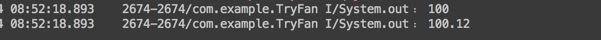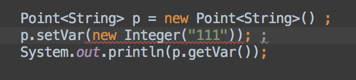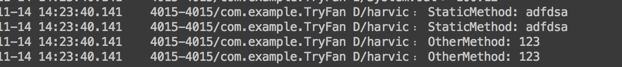# Java泛型详解：<T>和Class<T>的使用。泛型类，泛型方法的详细使用实例

## 一、引入

### 1、泛型是什么

[java] view plain
1. ArrayList<String> strList = new ArrayList<String>();
2. ArrayList<Integer> intList = new ArrayList<Integer>();
3. ArrayList<Double> doubleList = new ArrayList<Double>();

### 2、没有泛型会怎样

[java] view plain
1. //设置Integer类型的点坐标
2. class IntegerPoint{
3.     private Integer x ;       // 表示X坐标
4.     private Integer y ;       // 表示Y坐标
5.     public void setX(Integer x){
6.         this.x = x ;
7.     }
8.     public void setY(Integer y){
9.         this.y = y ;
10.     }
11.     public Integer getX(){
12.         return this.x ;
13.     }
14.     public Integer getY(){
15.         return this.y ;
16.     }
17. }
18. //设置Float类型的点坐标
19. class FloatPoint{
20.     private Float x ;       // 表示X坐标
21.     private Float y ;       // 表示Y坐标
22.     public void setX(Float x){
23.         this.x = x ;
24.     }
25.     public void setY(Float y){
26.         this.y = y ;
27.     }
28.     public Float getX(){
29.         return this.x ;
30.     }
31.     public Float getY(){
32.         return this.y ;
33.     }
34. }

[java] view plain
1. class ObjectPoint{
2.     private Object x ;
3.     private Object y ;
4.     public void setX(Object x){
5.         this.x = x ;
6.     }
7.     public void setY(Object y){
8.         this.y = y ;
9.     }
10.     public Object getX(){
11.         return this.x ;
12.     }
13.     public Object getY(){
14.         return this.y ;
15.     }
16. }

[java] view plain
1. ObjectPoint integerPoint = new ObjectPoint();
2. integerPoint.setX(new Integer(100));
3. Integer integerX=(Integer)integerPoint.getX();

[java] view plain
1. integerPoint.setX(new Integer(100));

[java] view plain
1. Integer integerX=(Integer)integerPoint.getX();

[java] view plain
1. ObjectPoint floatPoint = new ObjectPoint();
2. floatPoint.setX(new Float(100.12f));
3. Float floatX = (Float)floatPoint.getX();

[java] view plain
1. ObjectPoint floatPoint = new ObjectPoint();
2. floatPoint.setX(new Float(100.12f));
3. String floatX = (String)floatPoint.getX();

[java] view plain
1. String floatX = (String)floatPoint.getX();

## 二、各种泛型定义及使用

### 1、泛型类定义及使用

[java] view plain
1. //定义
2. class Point<T>{// 此处可以随便写标识符号
3.     private T x ;
4.     private T y ;
5.     public void setX(T x){//作为参数
6.         this.x = x ;
7.     }
8.     public void setY(T y){
9.         this.y = y ;
10.     }
11.     public T getX(){//作为返回值
12.         return this.x ;
13.     }
14.     public T getY(){
15.         return this.y ;
16.     }
17. };
18. //IntegerPoint使用
19. Point<Integer> p = new Point<Integer>() ;
20. p.setX(new Integer(100)) ;
21. System.out.println(p.getX());
22.
23. //FloatPoint使用
24. Point<Float> p = new Point<Float>() ;
25. p.setX(new Float(100.12f)) ;
26. System.out.println(p.getX());（1）、定义泛型：Point<T>

（2）类中使用泛型

[java] view plain
1. //定义变量
2. private T x ;
3. //作为返回值
4. public T getX(){
5.     return x ;
6. }
7. //作为参数
8. public void setX(T x){
9.     this.x = x ;
10. }
（3）使用泛型类

[java] view plain
1. //IntegerPoint使用
2. Point<Integer> p = new Point<Integer>() ;
3. p.setX(new Integer(100)) ;
4. System.out.println(p.getX());
5.
6. //FloatPoint使用
7. Point<Float> p = new Point<Float>() ;
8. p.setX(new Float(100.12f)) ;
9. System.out.println(p.getX());

[java] view plain
1. Point<String> p = new Point<String>() ;

[java] view plain
1. //IntegerPoint使用
2. Point<Integer> p = new Point<Integer>() ;
3. //FloatPoint使用
4. Point<Float> p = new Point<Float>() ;

[java] view plain
1. public class ArrayList<E>{
2.     …………
3. }

(4）使用泛型实现的优势

（1）、不用强制转换

[java] view plain
1. //使用Object作为返回值，要强制转换成指定类型
2. Float floatX = (Float)floatPoint.getX();
3. //使用泛型时，不用强制转换，直接出来就是String
4. System.out.println(p.getVar());
(2)、在settVar()时如果传入类型不对，编译时会报错### 2、多泛型变量定义及字母规范

（1）、多泛型变量定义

[java] view plain
1. class MorePoint<T,U>{
2. }

[java] view plain
1. class MorePoint<T,U,A,B,C>{
2. }

[java] view plain
1. class MorePoint<T,U> {
2.     private T x;
3.     private T y;
4.
5.     private U name;
6.
7.     public void setX(T x) {
8.         this.x = x;
9.     }
10.     public T getX() {
11.         return this.x;
12.     }
13.     …………
14.     public void setName(U name){
15.         this.name = name;
16.     }
17.
18.     public U getName() {
19.         return this.name;
20.     }
21. }
22. //使用
23. MorePoint<Integer,String> morePoint = new MorePoint<Integer, String>();
24. morePoint.setName("harvic");
25. Log.d(TAG, "morPont.getName:" + morePoint.getName());

（2）、字母规范

[java] view plain
1. class Point<T>{
2.  …………
3. }

•  E — Element，常用在java Collection里，如：List<E>,Iterator<E>,Set<E>
•  K,V — Key，Value，代表Map的键值对
•  N — Number，数字
•  T — Type，类型，如String，Integer等等

### 3、泛型接口定义及使用

[java] view plain
1. interface Info<T>{        // 在接口上定义泛型
2.     public T getVar() ; // 定义抽象方法，抽象方法的返回值就是泛型类型
3.     public void setVar(T x);
4. }

（1）、使用方法一：非泛型类

[java] view plain
1. class InfoImpl implements Info<String>{   // 定义泛型接口的子类
2.     private String var ;                // 定义属性
3.     public InfoImpl(String var){        // 通过构造方法设置属性内容
4.         this.setVar(var) ;
5.     }
6.     @Override
7.     public void setVar(String var){
8.         this.var = var ;
9.     }
10.     @Override
11.     public String getVar(){
12.         return this.var ;
13.     }
14. }
15.
16. public class GenericsDemo24{
17.     public  void main(String arsg[]){
18.         InfoImpl i = new InfoImpl("harvic");
19.         System.out.println(i.getVar()) ;
20.     }
21. };

[java] view plain
1. class InfoImpl implements Info<String>{
2.  …………
3. }

[java] view plain
1. public class GenericsDemo24{
2.     public  void main(String arsg[]){
3.         InfoImpl i = new InfoImpl("harvic");
4.         System.out.println(i.getVar()) ;
5.     }
6. };
（2）、使用方法二：泛型类

[java] view plain
1. interface Info<T>{        // 在接口上定义泛型
2.     public T getVar() ; // 定义抽象方法，抽象方法的返回值就是泛型类型
3.     public void setVar(T var);
4. }
5. class InfoImpl<T> implements Info<T>{   // 定义泛型接口的子类
6.     private T var ;             // 定义属性
7.     public InfoImpl(T var){     // 通过构造方法设置属性内容
8.         this.setVar(var) ;
9.     }
10.     public void setVar(T var){
11.         this.var = var ;
12.     }
13.     public T getVar(){
14.         return this.var ;
15.     }
16. }
17. public class GenericsDemo24{
18.     public static void main(String arsg[]){
19.         InfoImpl<String> i = new InfoImpl<String>("harvic");
20.         System.out.println(i.getVar()) ;
21.     }
22. };

[java] view plain
1. class InfoImpl<T> implements Info<T>{   // 定义泛型接口的子类
2.     private T var ;             // 定义属性
3.     public InfoImpl(T var){     // 通过构造方法设置属性内容
4.         this.setVar(var) ;
5.     }
6.     public void setVar(T var){
7.         this.var = var ;
8.     }
9.     public T getVar(){
10.         return this.var ;
11.     }
12. }

[java] view plain
1. public class GenericsDemo24{
2.     public static void main(String arsg[]){
3.         Info<String> i = new InfoImpl<String>("harvic");
4.         System.out.println(i.getVar()) ;
5.     }
6. };

[java] view plain
1. class InfoImpl<T,K,U> implements Info<U>{   // 定义泛型接口的子类
2.      private U var ;
3.      private T x;
4.      private K y;
5.      public InfoImpl(U var){        // 通过构造方法设置属性内容
6.          this.setVar(var) ;
7.      }
8.      public void setVar(U var){
9.          this.var = var ;
10.      }
11.      public U getVar(){
12.          return this.var ;
13.      }
14.  }

[java] view plain
1. public class GenericsDemo24{
2.     public  void main(String arsg[]){
3.         InfoImpl<Integer,Double,String> i = new InfoImpl<Integer,Double,String>("harvic");
4.         System.out.println(i.getVar()) ;
5.     }
6. }

### 4、泛型函数定义及使用

[java] view plain
1. public class StaticFans {
2.     //静态函数
3.     public static  <T> void StaticMethod(T a){
4.         Log.d("harvic","StaticMethod: "+a.toString());
5.     }
6.     //普通函数
7.     public  <T> void OtherMethod(T a){
8.         Log.d("harvic","OtherMethod: "+a.toString());
9.     }
10. }

[java] view plain
1. //静态方法
4.
5. //常规方法
6. StaticFans staticFans = new StaticFans();
7. staticFans.OtherMethod(new Integer(123));//使用方法一
8. staticFans.<Integer>OtherMethod(new Integer(123));//使用方法二[java] view plain

[java] view plain
1. StaticFans staticFans = new StaticFans();
2. staticFans.OtherMethod(new Integer(123));//使用方法一
3. staticFans.<Integer>OtherMethod(new Integer(123));//使用方法二

[java] view plain
1. public static <T> List<T> parseArray(String response,Class<T> object){
2.     List<T> modelList = JSON.parseArray(response, object);
3.     return modelList;
4. }

### 5、其它用法:Class<T>类传递及泛型数组

（1）、使用Class<T>传递泛型类Class对象

[java] view plain
1. public static List<SuccessModel> parseArray(String response){
2.     List<SuccessModel> modelList = JSON.parseArray(response, SuccessModel.class);
3.     return modelList;
4. }

[java] view plain
1. public class SuccessModel {
2.     private boolean success;
3.
4.     public boolean isSuccess() {
5.         return success;
6.     }
7.
8.     public void setSuccess(boolean success) {
9.         this.success = success;
10.     }
11. }

[java] view plain
1. public static List<SuccessModel> parseArray(String response){
2.     List<SuccessModel> modelList = JSON.parseArray(response, SuccessModel.class);
3.     return modelList;
4. }

[java] view plain
1. public static <T> List<T> parseArray(String response,Class<T> object){
2.     List<T> modelList = JSON.parseArray(response, object);
3.     return modelList;
4. }

[java] view plain
1. public final class Class<T> implements Serializable {
2.     …………
3. }

（2）、定义泛型数组

[java] view plain
1. //定义
2. public static <T> T[] fun1(T...arg){  // 接收可变参数
3.        return arg ;            // 返回泛型数组
4. }
5. //使用
6. public static void main(String args[]){
7.        Integer i[] = fun1(1,2,3,4,5,6) ;
8.        Integer[] result = fun1(i) ;
9. }

[java] view plain
1. public static <T> T[] fun1(T...arg){  // 接收可变参数
2.        return arg ;            // 返回泛型数组
3. }

# 下面是我自己实际使用泛型的几个实例。

## 关于泛型类的使用实例

import lombok.Data; @Datapublic class MultiObject<T> { 	/**     * 成功状态     */    private boolean success;     /**     * 异常     */    private Exception ex;     /**     * 数据     */    private T obj;		public MultiObject() {	} 	/**	 * 注意：当传入的泛型是Boolean时，就和第三个构造函数冲突了。	 */	public MultiObject(boolean success) {		this.success = success;	}		public MultiObject(Exception ex) {		this.success = false;		this.ex = ex;	}		public MultiObject(T value) {		this.success = true;		this.obj = value;	}}

1，成功与否。对应属性success。
2，异常信息。对应属性ex。若是操作正常执行，则就不在意这个属性的值。
3，我们操作的最终目的对象。对应属性obj。

## 关于泛型方法的使用实例

1，一个是泛型表示某一个类型的参数。为的传递某一类的参数对象
2，另一个则是传递的不是参数，而是代表Class，某一个类。

    /**     * 将Json字符串信息转换成对应的Java对象     *     * @param json json字符串对象     * @param c    对应的类型     */    public static <T> T parseJsonToObj(String json, Class<T> c) {        try {            JSONObject jsonObject = JSONObject.parseObject(json);            return JSON.toJavaObject(jsonObject, c);        } catch (Exception e) {            LOG.error(e.getMessage());        }        return null;    }

Collector collectorObj = JSONUtils.parseJsonToObj(collector, Collector.class);Flume flume = JSONUtils.parseJsonToObj(flumeJson, Flume.class);Probe probe = JSONUtils.parseJsonToObj(probeJson, Probe.class);

    /**     * @param dest   目的集合     * @param source 源集合     * @param <T>    集合参数的类型     */    private static <T> void listAddAllAvoidNPE(List<T> dest, List<T> source) {        if (source == null) {            return;        }        dest.addAll(source);    }     private static <T> void listAddAvoidNull(List<T> dest, T source) {        if (source == null) {            return;        }        dest.add(source);    }

	List<ProbeObject> list = Lists.newArrayList();	listAddAllAvoidNPE(list, decoder.getProperties());

posted @ 2018-11-08 14:10  星朝  阅读(86530)  评论(10编辑  收藏